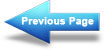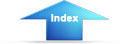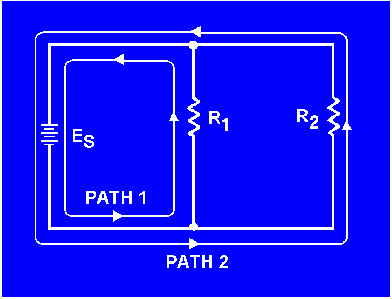PARALLEL CIRCUIT with which you must become familiar. Where the series circuit has only one path for current, the parallel circuit has more than one path for current."> Parallel DC circuitsCustom SearchPower transfer and efficiencyMatter, Energy, and ElectricityVoltage in a Parallel CircuitPARALLEL DC CIRCUITS

The discussion of electrical circuits presented up to this point has been concerned with series circuits in which there is only one path for current. There is another basic type of circuit known as the PARALLEL CIRCUIT with which you must become familiar. Where the series circuit has only one path for current, the parallel circuit has more than one path for current.

Ohm's law and Kirchhoff's law apply to all electrical circuits, but the characteristics of a parallel dc circuit are different than those of a series dc circuit.

PARALLEL CIRCUIT CHARACTERISTICS
A PARALLEL CIRCUIT is defined as one having more than one current path connected to a common voltage source. Parallel circuits, therefore, must contain two or more resistances which are not connected in series. An example of a basic parallel circuit is shown in figure 3-37.

Figure 3-37. - Example of a basic parallel circuit.Start at the voltage source (Es) and trace counterclockwise around the circuit. Two complete and separate paths can be identified in which current can flow. One path is traced from the source, through resistance R1, and back to the source. The other path is from the source, through resistance R2, and back to the source.Integrated Publishing, Inc. - A (SDVOSB) Service Disabled Veteran Owned Small Business